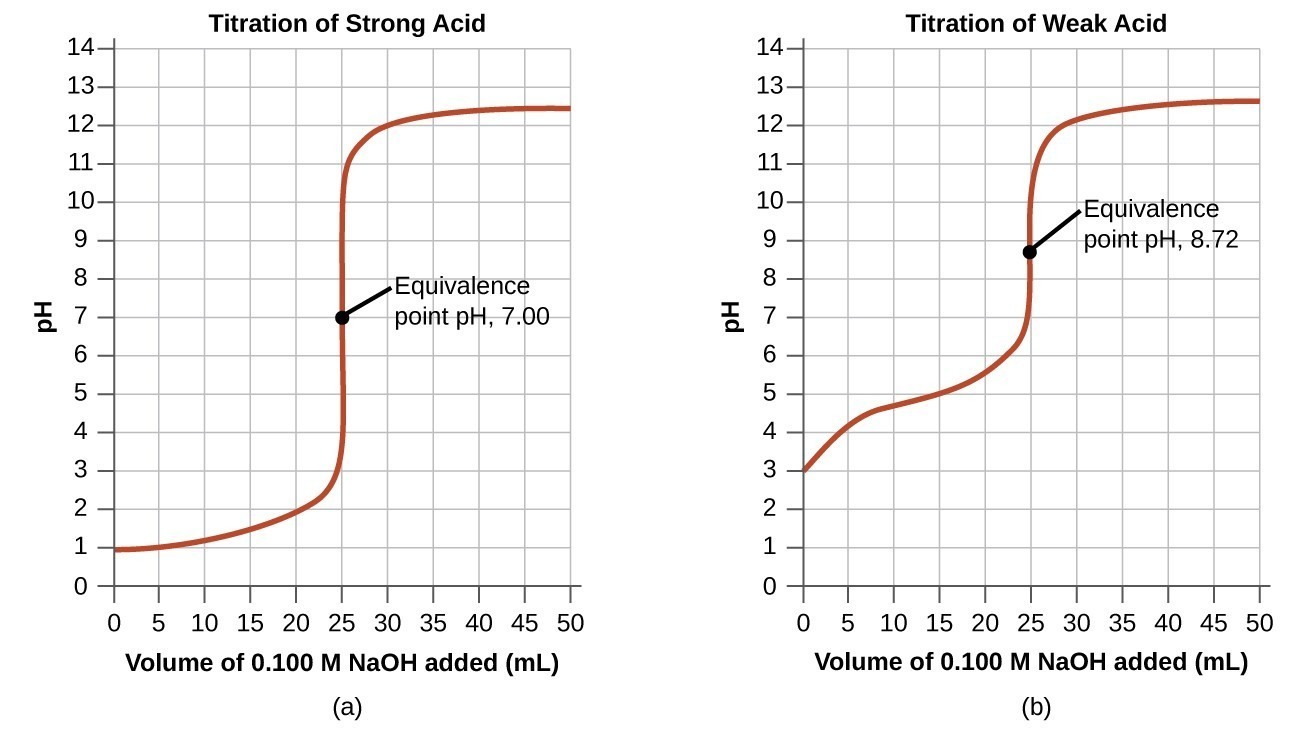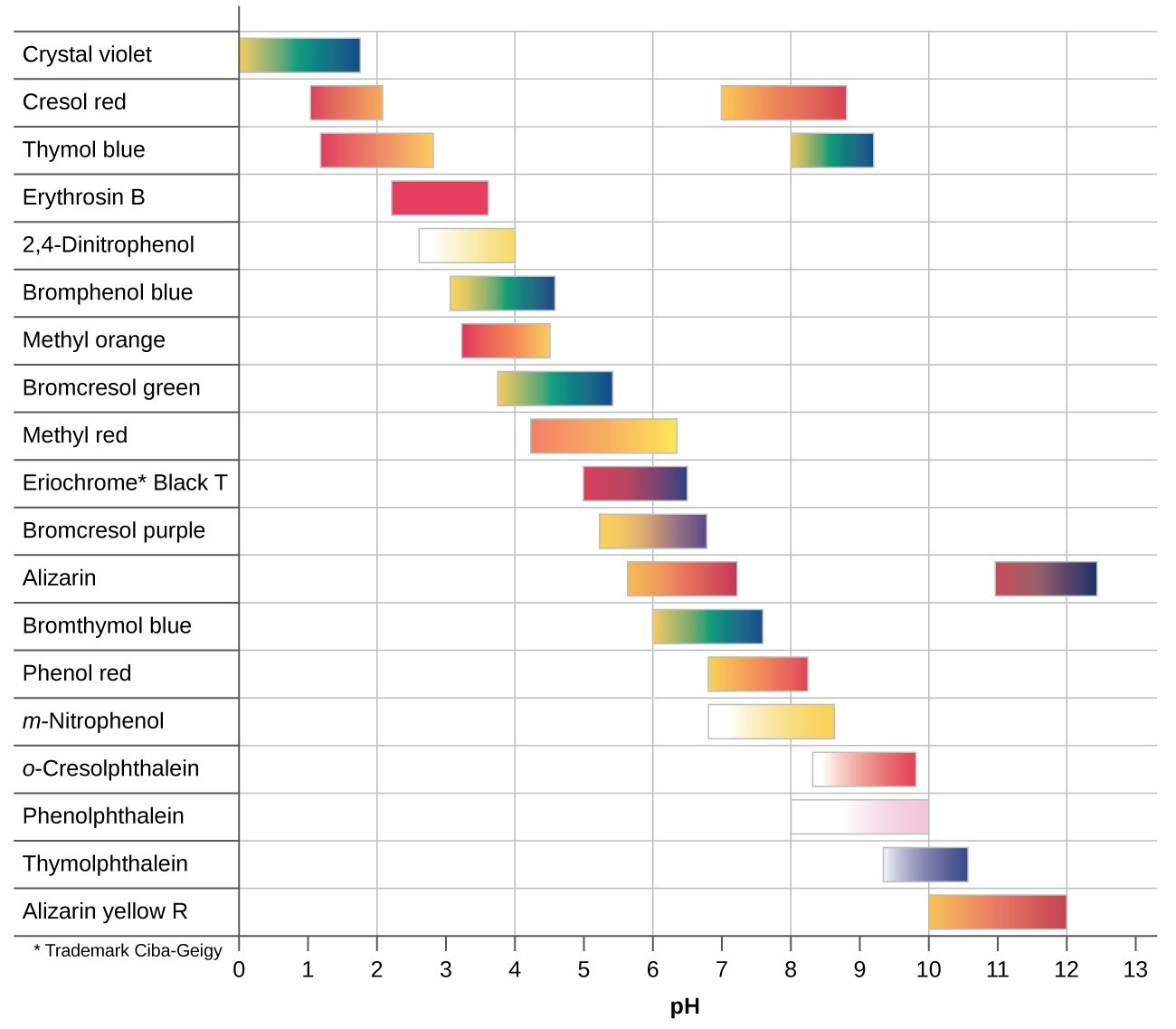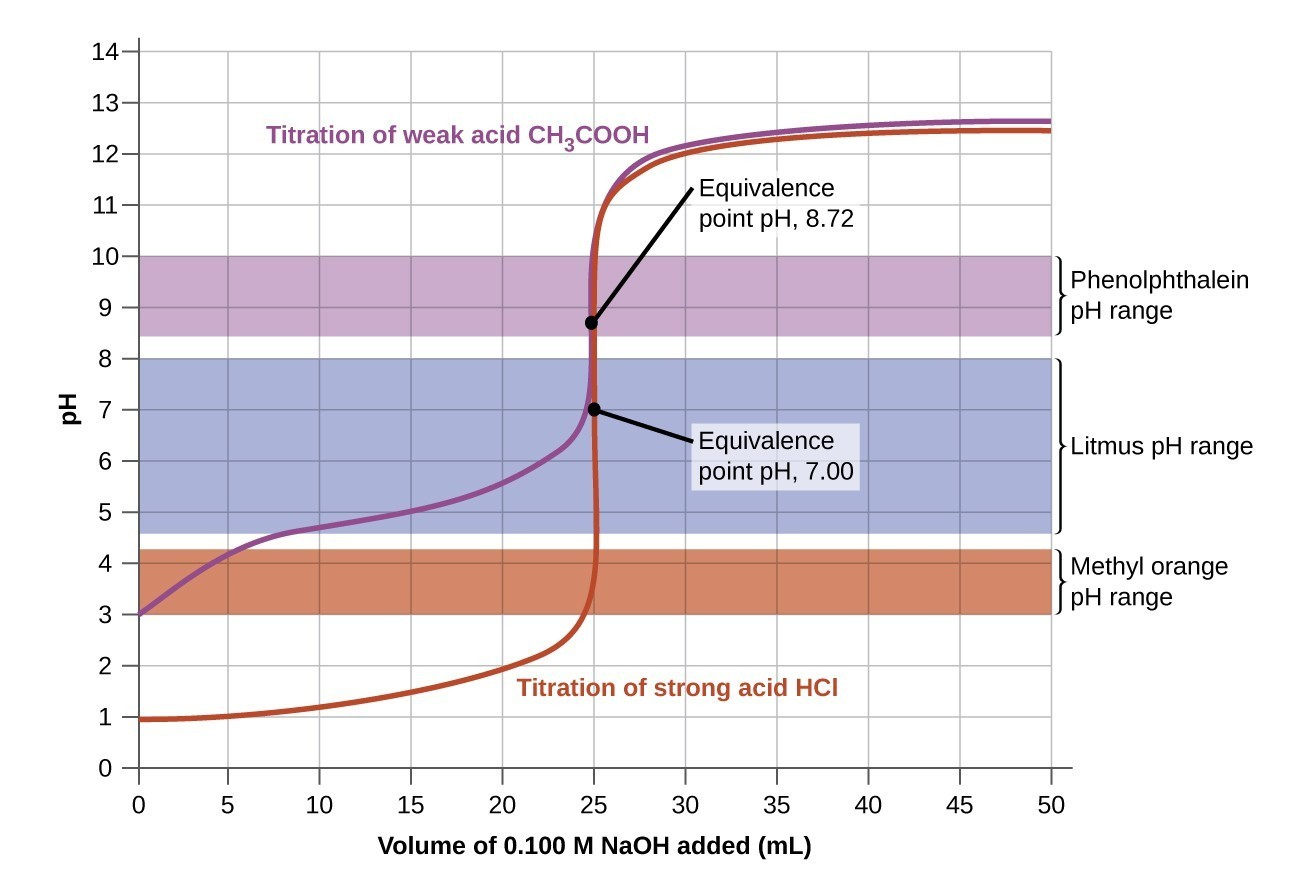## Acid-Base Titrations

### Learning Outcomes

• Interpret titration curves for strong and weak acid-base systems
• Compute sample pH at important stages of a titration
• Explain the function of acid-base indicators

As seen in the chapter on the stoichiometry of chemical reactions, titrations can be used to quantitatively analyze solutions for their acid or base concentrations. In this section, we will explore the underlying chemical equilibria that make acid-base titrimetry a useful analytical technique.

## Titration Curve

titration curve is a plot of some solution property versus the amount of added titrant. For acid-base titrations, solution pH is a useful property to monitor because it varies predictably with the solution composition and, therefore, may be used to monitor the titration’s progress and detect its end point. The following example exercise demonstrates the computation of pH for a titration solution after additions of several specified titrant volumes. The first example involves a strong acid titration that requires only stoichiometric calculations to derive the solution pH. The second example addresses a weak acid titration requiring equilibrium calculations.

### Example 1: Calculating pH for Titration Solutions: Strong Acid/Strong Base

A titration is carried out for 25.00 mL of 0.100 M HCl (strong acid) with 0.100 M of a strong base NaOH the titration curve is shown in Figure 1 (below). Calculate the pH at these volumes of added base solution:

1. 0.00 mL
2. 12.50 mL
3. 25.00 mL
4. 37.50 mL

#### Check Your Learning

Calculate the pH for the strong acid/strong base titration between 50.0 mL of 0.100 M HNO3(aq) and 0.200 M NaOH (titrant) at the listed volumes of added base: 0.00 mL, 15.0 mL, 25.0 mL, and 40.0 mL.

In Example 1, we calculated pH at four points during a titration. Table 1 shows a detailed sequence of changes in the pH of a strong acid and a weak acid in a titration with NaOH.

Table 1. pH Values in the Titrations of a Strong Acid with a Strong Base and of a Weak Acid with a Strong Base
Volume of 0.100 M NaOH Added (mL) Moles of NaOH Added pH Values 0.100 M HCl pH Values 0.100 M CH3CO2H
0.0 0.0 1.00 2.87
5.0 0.00050 1.18 4.14
10.0 0.00100 1.37 4.57
15.0 0.00150 1.60 4.92
20.0 0.00200 1.95 5.35
22.0 0.00220 2.20 5.61
24.0 0.00240 2.69 6.13
24.5 0.00245 3.00 6.44
24.9 0.00249 3.70 7.14
25.0 0.00250 7.00 8.72
25.1 0.00251 10.30 10.30
25.5 0.00255 11.00 11.00
26.0 0.00260 11.29 11.29
28.0 0.00280 11.75 11.75
30.0 0.00300 11.96 11.96
35.0 0.00350 12.22 12.22
40.0 0.00400 12.36 12.36
45.0 0.00450 12.46 12.46
50.0 0.00500 12.52 12.52

The simplest acid-base reactions are those of a strong acid with a strong base. Table 1 shows data for the titration of a 25.0-mL sample of 0.100 M hydrochloric acid with 0.100 M sodium hydroxide. The values of the pH measured after successive additions of small amounts of NaOH are listed in the first column of this table, and are graphed in Figure 1, in a form that is called a titration curve. The pH increases slowly at first, increases rapidly in the middle portion of the curve, and then increases slowly again. The point of inflection (located at the midpoint of the vertical part of the curve) is the equivalence point for the titration. It indicates when equivalent quantities of acid and base are present. For the titration of a strong acid with a strong base, the equivalence point occurs at a pH of 7.00 and the points on the titration curve can be calculated using solution stoichiometry (Table 1 and Figure 1).Figure 1. (a) The titration curve for the titration of 25.00 mL of 0.100 M CH3CO2H (weak acid) with 0.100 M NaOH (strong base) has an equivalence point of 7.00 pH. (b) The titration curve for the titration of 25.00 mL of 0.100 M HCl (strong acid) with 0.100 M NaOH (strong base) has an equivalence point of 8.72 pH.

The titration of a weak acid with a strong base (or of a weak base with a strong acid) is somewhat more complicated than that just discussed, but it follows the same general principles. Let us consider the titration of 25.0 mL of 0.100 M acetic acid (a weak acid) with 0.100 M sodium hydroxide and compare the titration curve with that of the strong acid. Table 1 gives the pH values during the titration, Figure 1 shows the titration curve.

Although the initial volume and molarity of the acids are the same, there are important differences between the two titration curves. The titration curve for the weak acid begins at a higher value (less acidic) and maintains higher pH values up to the equivalence point. This is because acetic acid is a weak acid, which is only partially ionized. The pH at the equivalence point is also higher (8.72 rather than 7.00) due to the hydrolysis of acetate, a weak base that raises the pH:

${\text{CH}}_{3}{\text{CO}}_{2}{}^{-}\left(aq\right)+{\text{H}}_{2}\text{O}\left(l\right)\rightleftharpoons {\text{CH}}_{3}{\text{CO}}_{2}\text{H}\left(l\right)+{\text{OH}}^{-}\left(aq\right)$

After the equivalence point, the two curves are identical because the pH is dependent on the excess of hydroxide ion in both cases.

### Example 2: Titration of a Weak Acid with a Strong Base

The titration curve shown in Figure 3 is for the titration of 25.00 mL of 0.100 M CH3CO2H with 0.100 M NaOH. The reaction can be represented as ${\text{CH}}_{3}{\text{CO}}_{2}\text{H}+{\text{OH}}^{-}\longrightarrow {\text{CH}}_{3}{\text{CO}}_{2}{}^{-}+{\text{H}}_{2}\text{O}$.

1. What is the initial pH before any amount of the NaOH solution has been added? Ka = 1.8 $\times$ 10−5 for CH3CO2H.
2. Find the pH after 25.00 mL of the NaOH solution have been added.
3. Find the pH after 12.50 mL of the NaOH solution has been added.
4. Find the pH after 37.50 mL of the NaOH solution has been added.

#### Check Your Learning

Calculate the pH for the weak acid/strong base titration between 50.0 mL of 0.100 M HCOOH(aq) (formic acid) and 0.200 M NaOH (titrant) at the listed volumes of added base: 0.00 mL, 15.0 mL, 25.0 mL, and 30.0 mL.

## Acid-Base Indicators

Certain organic substances change color in dilute solution when the hydronium ion concentration reaches a particular value. For example, phenolphthalein is a colorless substance in any aqueous solution with a hydronium ion concentration greater than 5.0 $\times$ 10−9M (pH < 8.3). In more basic solutions where the hydronium ion concentration is less than 5.0 $\times$ 10−9M (pH > 8.3), it is red or pink. Substances such as phenolphthalein, which can be used to determine the pH of a solution, are called acid-base indicators. Acid-base indicators are either weak organic acids or weak organic bases.

The equilibrium in a solution of the acid-base indicator methyl orange, a weak acid, can be represented by an equation in which we use HIn as a simple representation for the complex methyl orange molecule:

$\begin{array}{ccc}\text{HIn}\left(aq\right)+{\text{H}}_{2}\text{O}\left(l\right)& \rightleftharpoons & {\text{H}}_{3}{\text{O}}^{\text{+}}\left(aq\right)+{\text{In}}^{-}\left(aq\right)\\ \text{red}& &\text{yellow}\end{array}$

${K}_{a}=\frac{\left[{\text{H}}_{3}{\text{O}}^{\text{+}}\right]\left[{\text{In}}^{-}\right]}{\left[\text{HIn}\right]}=4.0\times {10}^{-4}$

The anion of methyl orange, In, is yellow, and the nonionized form, HIn, is red. When we add acid to a solution of methyl orange, the increased hydronium ion concentration shifts the equilibrium toward the nonionized red form, in accordance with Le Châtelier’s principle. If we add base, we shift the equilibrium towards the yellow form. This behavior is completely analogous to the action of buffers.

The perceived color of an indicator solution is determined by the ratio of the concentrations of the two species In and HIn. If most of the indicator (typically about 60−90% or more) is present as In, the perceived color of the solution is yellow. If most is present as HIn, then the solution color appears red. The Henderson-Hasselbalch equation is useful for understanding the relationship between the pH of an indicator solution and its composition (thus, perceived color):

$\text{pH} = \text{p}Ka + \text{log}(\frac{[\text{In}^-]}{[\text{HIn}]})$

In solutions where pH > pKa, the logarithmic term must be positive, indicating an excess of the conjugate base form of the indicator (yellow solution). When pH < pKa, the log term must be negative, indicating an excess of the conjugate acid (red solution). When the solution pH is close to the indicator pKa, appreciable amounts of both conjugate partners are present, and the solution color is that of an additive combination of each (yellow and red, yielding orange). The color change interval (or pH interval) for an acid-base indicator is defined as the range of pH values over which a change in color is observed, and for most indicators this range is approximately pKa ± 1.

There are many different acid-base indicators that cover a wide range of pH values and can be used to determine the approximate pH of an unknown solution by a process of elimination. Universal indicators and pH paper contain a mixture of indicators and exhibit different colors at different pHs. Figure 2 presents several indicators, their colors, and their color-change intervals.Figure 2. This chart illustrates the ranges of color change for several acid-base indicators.Figure 3. The graph shows a titration curve for the titration of 25.00 mL of 0.100 M CH3CO2H (weak acid) with 0.100 M NaOH (strong base) and the titration curve for the titration of HCl (strong acid) with NaOH (strong base). The pH ranges for the color change of phenolphthalein, litmus, and methyl orange are indicated by the shaded areas.

The titration curves shown in Figure 3 illustrate the choice of a suitable indicator for specific titrations. In the strong acid titration, use of any of the three indicators should yield reasonably sharp color changes and accurate end point determinations. For this titration, the solution pH reaches the lower limit of the methyl orange color change interval after addition of ~24 mL of titrant, at which point the initially red solution would begin to appear orange. When 25 mL of titrant has been added (the equivalence point), the pH is well above the upper limit and the solution will appear yellow. The titration’s end point may then be estimated as the volume of titrant that yields a distinct orange-to-yellow color change. This color change would be challenging for most human eyes to precisely discern. More-accurate estimates of the titration end point are possible using either litmus or phenolphthalein, both of which exhibit color change intervals that are encompassed by the steep rise in pH that occurs around the 25.00 mL equivalence point.

The weak acid titration curve in Figure 3 shows that only one of the three indicators is suitable for end point detection. If methyl orange is used in this titration, the solution will undergo a gradual red-to-orange-to-yellow color change over a relatively large volume interval (0–6 mL), completing the color change well before the equivalence point (25 mL) has been reached. Use of litmus would show a color change that begins after adding 7–8 mL of titrant and ends just before the equivalence point. Phenolphthalein, on the other hand, exhibits a color change interval that nicely brackets the abrupt change in pH occurring at the titration’s equivalence point. A sharp color change from colorless to pink will be observed within a very small volume interval around the equivalence point.

### Key Concepts and Summary

The titration curve for an acid-base titration is typically a plot of pH versus volume of added titrant. These curves are useful in selecting appropriate acid-base indicators that will permit accurate determinations of titration end points.

### Try It

1. Explain how to choose the appropriate acid-base indicator for the titration of a weak base with a strong acid.
2. Explain why an acid-base indicator changes color over a range of pH values rather than at a specific pH.
3. Why can we ignore the contribution of water to the concentrations of ${\text{H}}_{3}{\text{O}}^{\text{+}}$ in the solutions of following acids: (1) 0.0092 M HClO, a weak acid, (2) 0.0810 M HCN, a weak acid, and (3) 0.120 M $\text{Fe}{\left({\text{H}}_{2}\text{O}\right)}_{6}{}^{2+}$ a weak acid, Ka = 1.6 $\times$ 10−7, but not the contribution of water to the concentration of OH?
4. We can ignore the contribution of water to the concentration of OH in a solution of the following bases (1) 0.0784 M C6H5NH2, a weak base and (2) 0.11 M (CH3)3N, a weak base, but not the contribution of water to the concentration of ${\text{H}}_{3}{\text{O}}^{\text{+}}?$
5. Draw a curve similar to that shown in Figure 3 for a series of solutions of NH3. Plot [OH] on the vertical axis and the total concentration of NH3 (both ionized and nonionized NH3 molecules) on the horizontal axis. Let the total concentration of NH3 vary from 1 $\times$ 10−10M to 1 $\times$ 10−2M.
6. The indicator dinitrophenol is an acid with a Ka of 1.1 $\times$ 10−4. In a 1.0 $\times$ 10−4M solution, it is colorless in acid and yellow in base. Calculate the pH range over which it goes from 10% ionized (colorless) to 90% ionized (yellow).

### Try It

Draw a curve for a series of solutions of HF. Plot ${\left[{\text{H}}_{3}{\text{O}}^{\text{+}}\right]}_{\text{total}}$ on the vertical axis and the total concentration of HF (the sum of the concentrations of both the ionized and nonionized HF molecules) on the horizontal axis. Let the total concentration of HF vary from 1 $\times$ 10−10M to 1 $\times$ 10−2M.

#### Question 2

Calculate the pH at the following points in a titration of 40 mL (0.040 L) of 0.100 M barbituric acid (Ka = 9.8 $\times$ 10−5) with 0.100 M KOH.

1. no KOH added
2. 20 mL of KOH solution added
3. 39 mL of KOH solution added
4. 40 mL of KOH solution added
5. 41 mL of KOH solution added

## Glossary

acid-base indicator: organic acid or base whose color changes depending on the pH of the solution it is in

color-change interval: range in pH over which the color change of an indicator takes place

titration curve: plot of the pH of a solution of acid or base versus the volume of base or acid added during a titration

1. Titration of 25.00 mL of 0.100 M HCl (0.00250 mol of HCI) with 0.100 M NaOH.
2. Titration of 25.00 mL of 0.100 M CH3CO2H (0.00250 mol of CH3CO2H) with 0.100 M NaOH.